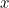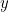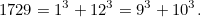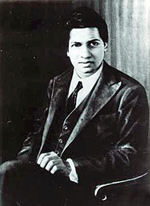# Mystery number: SolutionFind the numberthat satisfies these two properties:

• The digits ofadd up to a numberwhereequalstimes the number you get when you reverse the digits of.
• Reverse the digits ofand find the prime factors of the number you get. Then take the sum of the squares of these prime factors and halve it. Removing the digit 0 from the new number yields back.

### Solution

The answer is 1729. We've chosen this puzzle in honour of the Indian mathematician Srinivasa Ramanujan who would have turned 125 on 22nd December 2012. The number is known as the Hardy-Ramanujan number after Ramanujan and the mathematician and Godfrey Hardy. It has another interesting property: you can write it as a sum of cubes in two different ways:Srinivasa Ramanujan, 1887-1920. Image: Konrad Jacobs.

Hardy told the following story: "I remember once going to see [Ramanujan] when he was ill at Putney. I had ridden in taxi cab number 1729 and remarked that the number seemed to me rather a dull one, and that I hoped it was not an unfavorable omen. 'No,' he replied, 'it is a very interesting number; it is the smallest number expressible as the sum of two cubes in two different ways.' "

You can read more about Ramanujan and the fascinating story of his genius in A disappearing number.